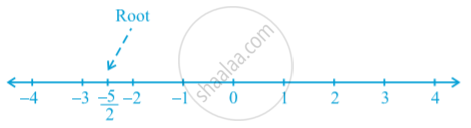Share

# Concept of Linear Equations in Two Variables

#### notes

Consider the following equation: 2x + 5 = 0
the root of the equation, is  -5/2. This can be represented on the number line as shown below:While solving an equation, you must always keep the following points in mind:
The solution of a linear equation is not affected when:
(i) the same number is added to (or subtracted from) both the sides of the equation.
(ii) you multiply or divide both the sides of the equation by the same non-zero number.
So, any equation which can be put in the form ax + by + c = 0, where a, b and c are real numbers, and a and b are not both zero, is called a linear equation in two variables.

### Shaalaa.com

Linear Equation in 2 variables part 1 [00:11:03]
S
0%

S